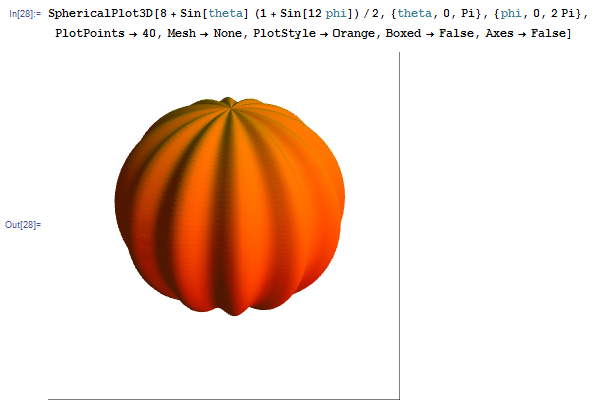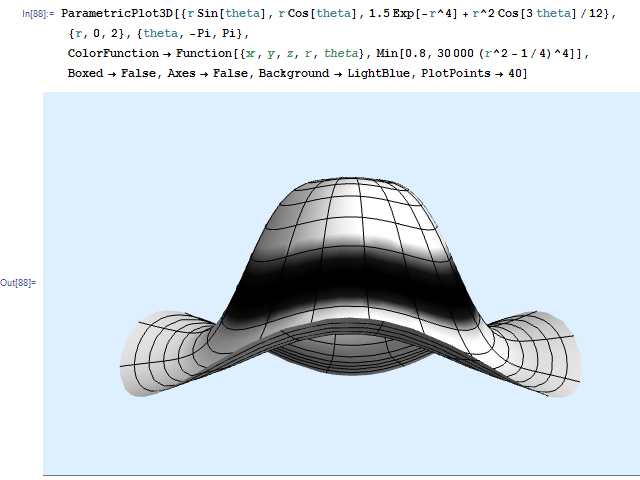# Surfaces

Many natural objects can be modelled by startlingly simple equations. For instance, there is a sextic surface (degree-6 polynomial equation in three variables) which resembles a heart. As such, it has been nicknamed the ‘heart surface’:Now that we’re approaching Hallowe’en, I decided to do a similar thing to create a pumpkin. Due to its approximate spherical shape, the spherical coordinate system seemed like a good idea. I then used trigonometric functions to create the undulations:Similarly, a cylindrical coordinate system is perfect for modelling surfaces such as hats. This time, I attempted to recreate an infamous hat worn by certain students at the Part IA lectures in the Cambridge Mathematical Tripos:If you can decipher the Mathematica code, you’ll see that the equation (in cylindrical coordinates) is z = (3/2) Exp[-r^4] + (1/12) r^2 Cos[3 theta]. This was derived by modifying the ordinary Gaussian distribution until it had the correct shape. The trigonometric term causes the rim of the hat to vary sinusoidally. I then coloured the hat depending on radius to give the appearance of the black band.

This entry was posted in Uncategorized. Bookmark the permalink.

### 0 Responses to Surfaces

1.johnicholas says:

Hal Abelson wrote a book called “Turtle Geometry” which focuses on the characterization of shapes from the perspective of a Logo turtle drawing them. Presumably, the pumpkin and to a lesser extent the hat have similar local, generative rules to them – for example, the ripple at the edge of a hat (or some leaves) probably is caused by local patches having “too much area for their circumference”. Hats are often made using forms, though, which would argue against a local, generative rule. The form might be turned on a lathe, which would impose some symmetry to it, but the profile could be completely arbitrary.

However, the pumpkin’s rule for “how to become pumpkin-shaped” are probably even simpler than these equations, though it might need a morphogenesis-specific vocabulary to see the simplicity.

•apgoucher says:

Yes, turtle geometry is rather interesting, especially when used in conjunction with L-systems to generate recursive patterns (some simple fractals can be defined in this way).

As for specifying a hat based on local curvature, this is indeed possible. I think that crinkly leaves and the brim of the hat are actually hyperbolic, i.e. too much circumference for the area, rather than the other way around. People have crocheted hyperbolic planes in precisely this way; I believe I included a picture of a hyperbolic crocheted coral reef on an early CP4space posting.

Morphogenesis is very complicated, and it is astonishing that something so complex as a human being, for instance, can be specified by a genome of three billion base pairs (equivalent to 750 megabytes — roughly the contents of a CD).